# How to quickly calculate the square root of a value in Excel?

In this article, users can quickly calculate the square root of a value in Excel. With the help of SQRT() function, the manual computation of deriving the square root of large numbers may be eliminated and save the user time. Two examples will be illustrated; both the provided examples calculate the square root provided for the specified value. The first example uses the user-defined formula to solve the provided task. The user needs to viably provide the proper address of the cell inside the SQRT() function to generate an accurate result. The second example uses the Kutools.

## Example 1: To calculate the square root of provided values by using the system provided formula.

### Step 1

To understand the process of evaluating the square root of a value in Excel. Users need to create a table as highlighted in the below figure.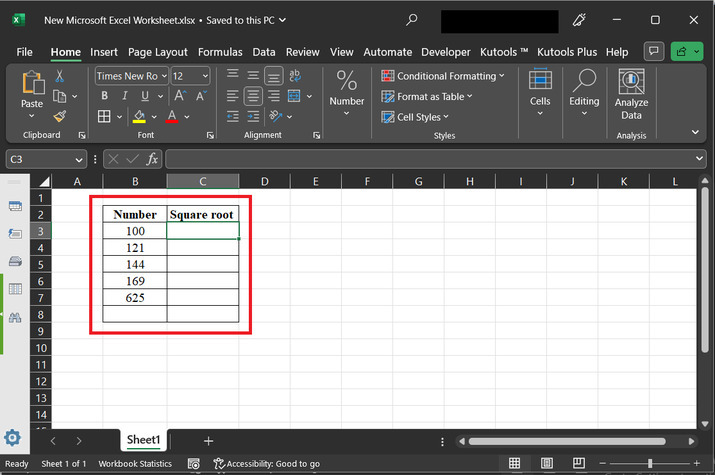### Step 2

To apply the formula properly click on the C3 cell, and type formula “=SQRT(B3)”. Consider the below-depicted image for reference −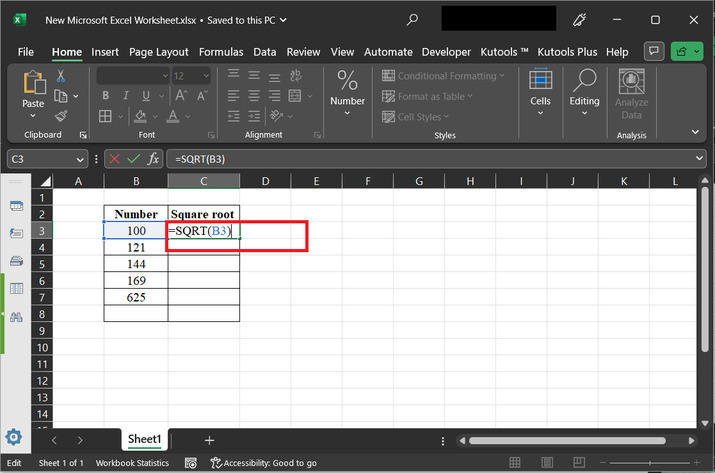Explanation for “=SQRT(B3)” formula

• The formula SQRT(B3) is a mathematical expression that calculates the square root of the value in cell B3. In simpler terms, it finds the number that, when multiplied by itself, gives the value in cell B3.

• Let's understand this with an example. Suppose cell B3 contains the value 49. When you use the formula SQRT(B3), it will calculate the square root of 49, which is 7. This means that if you multiply 7 by itself (7 * 7), you will get the value 49.

• Similarly, if B3 contains the value 36, applying the formula SQRT(B3) will give you the square root of 36, which is 6. This means that 6 multiplied by itself (6 * 6) equals 36.

• The SQRT function is widely used in various fields, such as mathematics, physics, engineering, and finance.

### Step 3

Press the “Enter” key to display the data. Consider the below-provided results −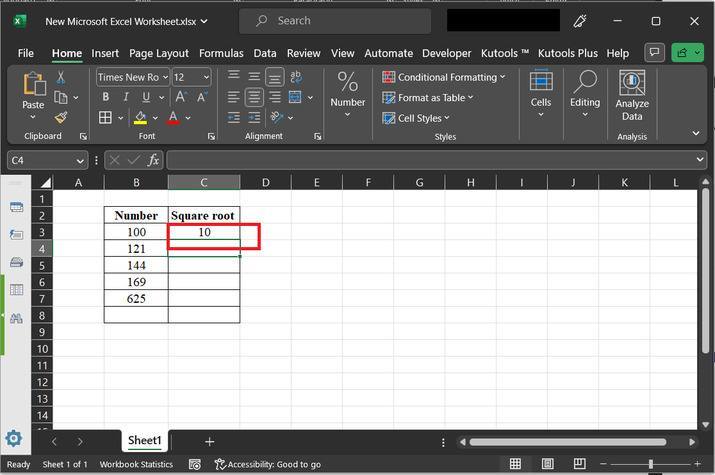### Step 4

Drag the fill handle. This step will allow the user to copy the formula, for different values to different rows.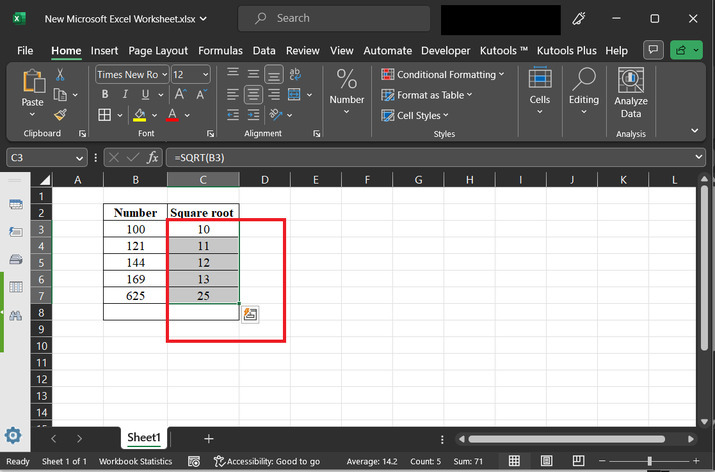## Example 2: To calculate the square root of provided values by using the kutools.

### Step 1

Consider an Excel sheet that contains a few numbers. These numbers will be converted to the associated square root by using the kutools.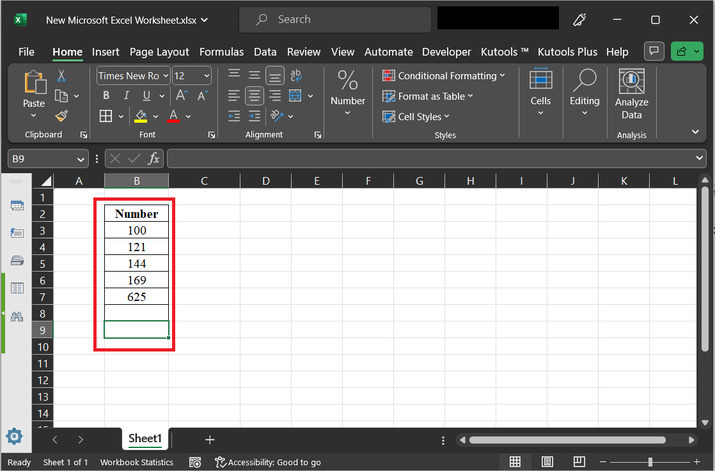### Step 2

To do so, click on the “Kutools” tab, and then select the option “Editing” option, and further select the option “More”. Then click on the “Operations…” options. As depicted below −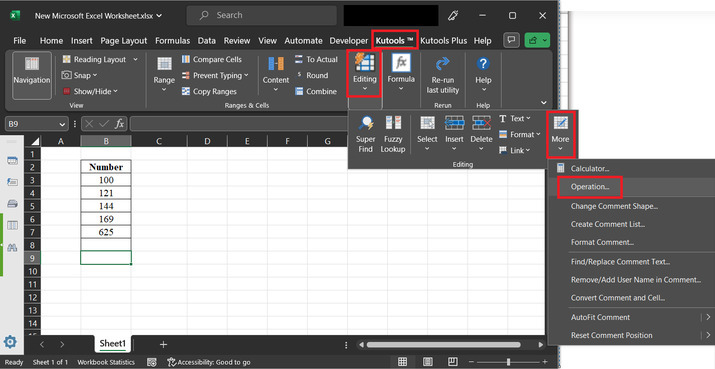### Step 3

The above step will display an operation tools dialog box. In the opened dialog box, under the operation label, select the option for “Function”. After that in the drop-down menu option click on the “SQRT” option. After that select the range from the Excel sheet to apply the provided function. The preview for the selected range will be displayed in the preview section. Refer to that preview, and if data values seem to be fine, then click on the “OK” button.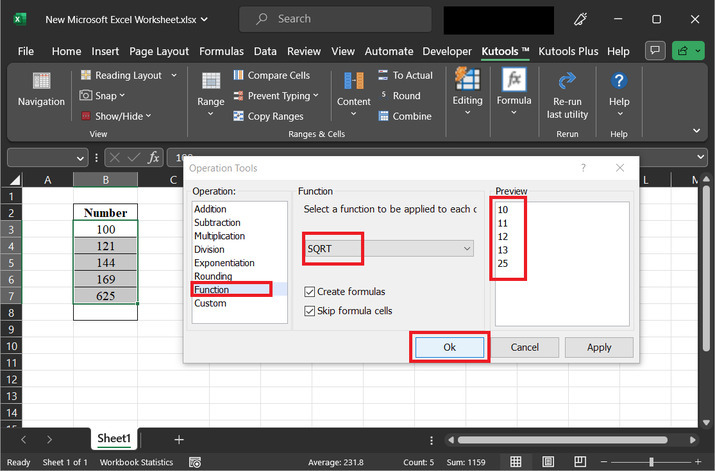### Step 4

This option will display the square root values. The final output snapshot is provided below −## Conclusion

Additionally, the flexibility of Excel allows you to apply the square root calculation across multiple cells or incorporate it into larger formulas and functions. Whether you are working on financial models, statistical analysis, or scientific research, Excel's efficient square root calculation capabilities help streamline your work and enhance your productivity. Mastering this feature will empower you to handle complex calculations with ease and unlock the full potential of Excel as a versatile tool for data analysis and computation.

Updated on: 29-Aug-2023

28 Views